A rough vertical board has an acceleration ‘a’ so that a 2 kg block pressing against it does not fall. The coefficient of friction between the block and the board should be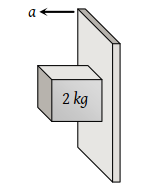(1) > g/a

(2) < g/a

(3) = g/a

(4) > a/g

High Yielding Test Series + Question Bank - NEET 2020

Difficulty Level:

A mass of 100g strikes the wall with speed 5m/s at an angle as shown in figure and it rebounds with the same speed. If the contact time is $2×{10}^{-3}\text{\hspace{0.17em}}\mathrm{sec}$, what is the force applied on the mass by the wall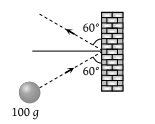(1) $250\sqrt{3}$N to right

(2) 250 N to right

(3) $250\sqrt{3}$N to left

(4) 250 N to left

Concept Questions :-

Application of laws
High Yielding Test Series + Question Bank - NEET 2020

Difficulty Level:

A lead ball strikes a wall and falls down, a tennis ball having the same mass and velocity strikes the wall and bounces back. Check the correct statement

(1) The momentum of the lead ball is greater than that of the tennis ball

(2) The lead ball suffers a greater change in momentum compared with the tennis ball

(3) The tennis ball suffers a greater change in momentum as compared with the lead ball

(4) Both suffer an equal change in momentum

Concept Questions :-

Application of laws
High Yielding Test Series + Question Bank - NEET 2020

Difficulty Level:

A ball of weight 0.1 kg coming with speed 30 m/s strikes with a bat and returns in opposite direction with speed 40 m/s, then the impulse is (Taking final velocity as positive)

(1) $-0.1×\left(40\right)-0.1×\left(30\right)$

(2) $0.1×\left(40\right)-0.1×\left(-30\right)$

(3) $0.1×\left(40\right)+0.1×\left(-30\right)$

(4) $0.1×\left(40\right)-0.1×\left(20\right)$

Concept Questions :-

Application of laws
High Yielding Test Series + Question Bank - NEET 2020

Difficulty Level:

A ball of mass m falls vertically to the ground from a height h1 and rebound to a height h2. The change in momentum of the ball on striking the ground is

(1) $mg\left({h}_{1}-{h}_{2}\right)$

(2) $m\left(\sqrt{2g{h}_{1}}+\sqrt{2g{h}_{2}}\right)$

(3) $m\sqrt{2g\left({h}_{1}+{h}_{2}\right)}$

(4) $m\sqrt{2g}\left({h}_{1}+{h}_{2}\right)$

Concept Questions :-

Application of laws
High Yielding Test Series + Question Bank - NEET 2020

Difficulty Level:

A force-time graph for a linear motion is shown in figure where the segments are circular. The linear momentum gained between zero and 8 second is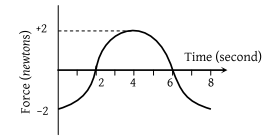1. $-2\pi \text{\hspace{0.17em}}newton×second$

2. $Zero\text{\hspace{0.17em}}newton×second$

3. $+4\pi \text{\hspace{0.17em}}newton×second$

4. $-6\pi \text{\hspace{0.17em}}newton×second$

Concept Questions :-

Newton laws
High Yielding Test Series + Question Bank - NEET 2020

Difficulty Level: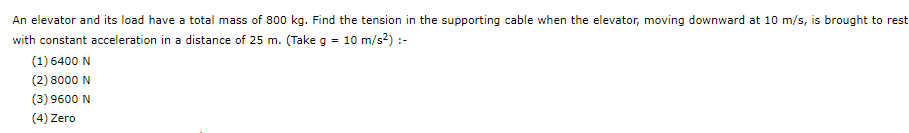Concept Questions :-

Application of laws

Difficulty Level:

Block A weighing 100 kg rests on a block B and is tied with a horizontal string to the wall at C. Block B weighs 200 kg. The coefficient of friction between A and B is 0.25 and between B and the surface is 1/3. The horizontal force P necessary to move the block B should be (g = 10 m/s2)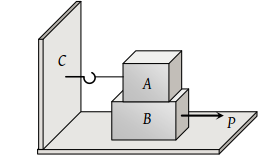(1) 1150 N

(2) 1250 N

(3) 1300 N

(4) 1420 N

High Yielding Test Series + Question Bank - NEET 2020

Difficulty Level:

A body of weight 50 N placed on a horizontal surface is just moved by a force of 28.2 N. The frictional force and the normal reaction are(1) 10 N, 15 N

(2) 20 N, 30 N

(3) 2 N, 3 N

(4) 5 N, 6 N

High Yielding Test Series + Question Bank - NEET 2020

Difficulty Level:

A 40 kg slab rests on a frictionless floor as shown in the figure. A 10 kg block rests on the top of the slab. The static coefficient of friction between the block and slab is 0.60 while the kinetic friction is 0.40. The 10 kg block is acted upon by a horizontal force 100 N. If g = 9.8 m/s2, the resulting acceleration of the slab will be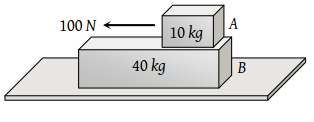(1) 0.98 m/s2

(2) 1.47 m/s2

(3) 1.52 m/s2

(4) 6.1 m/s2